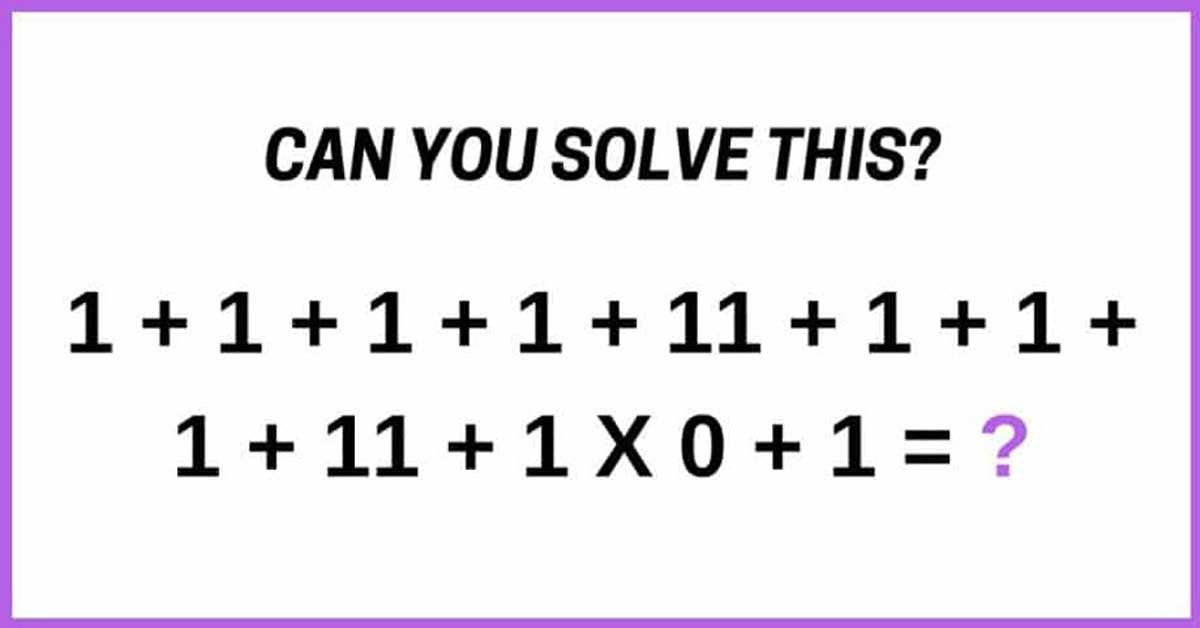# Everyone’s Arguing Over The Answer To This Simple Equation.## Take a look and see if you can solve it, then scroll down for the answer.Keep scrolling!

Just a little more, we wanna give you time to figure it out on your own!

Get it yet?## The correct answer is 30.

To arrive at this solution, you’ve got to use PEMDAS, which means you’ll do the multiplication first. The equation would be:

1 + 1 + 1 + 1 + 11 + 1 + 1 + 1 + 11 + (1 x 0) + 1

= 29 + 0 + 1

= 30

=====

Eyes Test: Spot The ODD One Out In Seconds. Can You?

## Easy task for you: Find the odd one out!Scroll down.

A

.

N

.

S

.

W

.

E

.

R